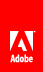# Number functions

This section describes the number functions that are available in expressions.

## ceiling

Returns the smallest integer value that is not less than a given numeric value. Values are smaller in the direction of negative infinity.

The ceiling function returns the smallest (closest to negative infinity) number that is not less than the argument and that is an integer.

#### Syntax

`    ceiling(Expression)`

#### Parameters

Expression is an expression that returns a numeric value.

#### Returns

An integer value.

## floor

Returns the largest integer value that is not greater than a given numeric value. Values are larger in the direction of positive infinity.

#### Syntax

`    floor(number)`

#### Parameters

number is a numeric value.

#### Returns

An integer value.

## number

The number function converts a given numeric value to a number.

#### Syntax

`    number(object?)`

#### Parameters

Zero or one objects.

A number value.

## round

Returns a whole number that is closest to a given numeric value.

#### Syntax

`    round(number)`

#### Parameters

number is any numeric value:

• If number is NaN, NaN is returned.

• If number is positive infinity, positive infinity is returned.

• If number is negative infinity, negative infinity is returned.

• If number is positive zero, positive zero is returned.

• If number is negative zero, negative zero is returned.

• If number is less than zero and greater than or equal to -0.5, negative zero is returned.

• If number is an equal distance from two different numbers, the number that is closest to positive infinity is returned.

#### Returns

An integer value.// Ethnio survey code removed